# Polar moment of inertia facts for kids

Kids Encyclopedia Facts
  Note: Different disciplines use the term moment of inertia to refer to different moments.  In physics, moment of inertia is strictly the second moment of mass with respect to distance from an axis, which characterizes an object's angular acceleration due to an applied torque.  In engineering (especially mechanical and civil), moment of inertia commonly refers to the second moment of the area.  When reading polar moment of inertia take care to verify that it is referring to "polar second moment of area" and not moment of inertia.  Polar second moment of area will have units of length to the fourth power (e.g.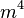$m^4$ or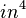$in^4$), while moment of inertia is mass times length squared (e.g.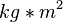$kg*m^2$ or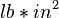$lb*in^2$).


The polar second moment of area (also referred to as "polar moment of inertia") is a measure of an object's ability to resist torsion as a function of its shape. It is one aspect of the second moment of area linked through the perpendicular axis theorem where the planar second moment of area uses a beam's cross-sectional shape to describe its resistance to deformation (bending) when subjected to a force applied in a plane parallel to its neutral axis, the polar second moment of area uses a beam's cross-sectional shape to describe its resistance to deformation (torsion) when a moment (torque) is applied in a plane perpendicular to the beam's neutral axis. While the planar second moment of area is most often denoted by the letter,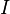$I$, the polar second moment of area is most often denoted by either,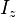$I_z$, or the letter,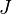$J$, in engineering textbooks.

The calculated values for the polar second moment of area are most often used describe a solid or hollow cylindrical shaft's resistance to torsion, as in a vehicle's axle or drive shaft. When applied to non-cylindrical beams or shafts, the calculations for the polar second moment of area becomes erroneous due to warping of the shaft/beam. In these instances, a torsional constant should be used, where a correctional constant is added to the value's calculation.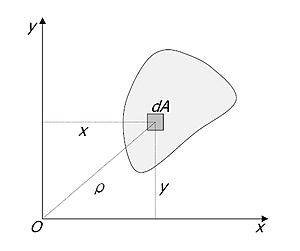A schematic showing how the polar second moment of area ("Polar Moment of Inertia") is calculated for an arbitrary shape of area, R, about an axis o, where ρ is the radial distance to the element dA.

The polar second moment of area carries the units of length to the fourth power (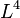$L^4$); meters to the fourth power ($m^4$) in the metric unit system, and inches to the fourth power ($in^4$) in the imperial unit system. The mathematical formula for direct calculation is given as a multiple integral over a shape's area,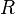$R$, at a distance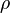$\rho$ from an arbitrary axis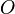$O$.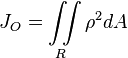$J_{O} = \iint\limits_R \rho^2 dA$.

In the most simple form, the polar second moment of area is a summation of the two planar second moments of area,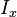$I_x$ and$I_y$. Using the Pythagorean theorem, the distance from axis$O$,$\rho$, can be broken into its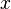$x$ and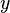$y$ components, and the change in area,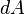$dA$, broken into its$x$ and$y$ components,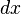$dx$ and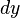$dy$.

Given the two formulas for the planar second moments of area: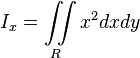$I_x = \iint\limits_R x^2 dxdy$, and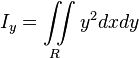$I_y = \iint\limits_R y^2 dxdy$

The relation to the polar second moment of area can be shown as:$J_{O} = \iint\limits_R \rho^2 dA$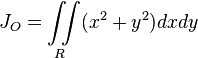$J_{O} = \iint\limits_R (x^2+y^2) dx dy$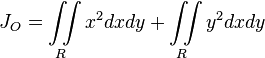$J_{O} = \iint\limits_R x^2 dxdy + \iint\limits_R y^2 dxdy$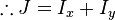$\therefore J = I_x + I_y$

In essence, as the magnitude of the polar second moment of area increases (i.e. large object cross-sectional shape), more torque will be required to cause a torsional deflection of the object. However, it must be noted that this does not have any bearing on the torsional rigidity provided to an object by its constituent materials; the polar second moment of area is simply rigidity provided to an object by its shape alone. Torsional rigidity provided by material characteristics is known as the shear modulus,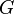$G$. Linking these two components of rigidity, one can calculate the angle of twist of a beam,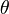$\theta$, using: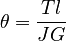$\theta = \frac{Tl}{JG}$

Where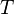$T$ is the applied moment (torque) and$l$ is the length of the beam. As shown, higher torques and beam lengths lead to higher angular deflections, where higher values for the polar second moment of area,$J$, and material shear modulus,$G$, reduces the potential for angular deflections.

## Related pages

• Moment (physics)
• Second moment of area
• List of second moments of area for standard shapes
• Shear modulus

## Images for kidsPolar moment of inertia Facts for Kids. Kiddle Encyclopedia.